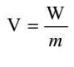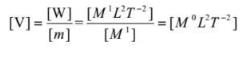Courses

# Test: Gravitational Potential Energy

## 20 Questions MCQ Test Physics Class 11 | Test: Gravitational Potential Energy

Description
This mock test of Test: Gravitational Potential Energy for Class 11 helps you for every Class 11 entrance exam. This contains 20 Multiple Choice Questions for Class 11 Test: Gravitational Potential Energy (mcq) to study with solutions a complete question bank. The solved questions answers in this Test: Gravitational Potential Energy quiz give you a good mix of easy questions and tough questions. Class 11 students definitely take this Test: Gravitational Potential Energy exercise for a better result in the exam. You can find other Test: Gravitational Potential Energy extra questions, long questions & short questions for Class 11 on EduRev as well by searching above.
QUESTION: 1

### Weight is

Solution:

Weight is a force caused by gravity. The weight of an object is the gravitational force between the object and the Earth. The more mass the object has the greater its weight will be. Weight is a force, so it's measured in newtons.

QUESTION: 2

### When a satellite moves in a circular orbit, the _______acceleration is provided by the gravitational attraction of the earth

Solution:

When any body or particle moves in circular orbit centripetal force acts on it and to move the object centripetal acceleration is necessary. So, when a satellite moves in circular orbit the centripetal acceleration is provided by the gravitational attraction of the earth.

QUESTION: 3

### An unmanned space probe is to the thrown with such a velocity that it does not return to earth. It should be thrown with

Solution:

It should be thrown with a velocity greater than or equal to escape velocity of the planet only then it will not return to Earth
Escape Velocity = √(2GM/R) = (2GM/R)1/2
Hence A is the correct answer.

QUESTION: 4

What could be the maximum value for gravitational potential energy?

Solution:

As, U=−Gm1m2/r,
it can be used to describe the potential energy in a system of point charges (or radially symmetric spheres) as a function of their separation distance r, then the maximum value is zero at infinite separation.
Hence, its maximum value is zero at infinity.

QUESTION: 5

The value of escape velocity on earth when the body is thrown making an angle of 45o with the horizontal is

Solution:

Escape velocity does not depend on the angle of projection. Escape velocity will remain the same.
Hence, escape velocity is 11.2km/s .

QUESTION: 6

The escape velocity for the moon is nearly

Solution:

In common usage, the initial point is on the surface of a planet or moon. On the surface of the Earth, the escape velocity is about 11.2 km/s, which is approximately 33 times the speed of sound (Mach 33) and several times the muzzle velocity of a rifle bullet (up to 1.7 km/s).

QUESTION: 7

A body is projected with velocity ‘v’ from a planet with escape velocity ‘ve‘ .What will happen if v < ve

Solution:

If velocity of satellite is is less than tge escape velocity then the satellite can go to certain hight and then cames back because the satellite is under the gravitational influence. therefore gravitational force acting on the satellite and due to this force satellite camse back

QUESTION: 8

If r represents the radius of the orbit of the satellite of mass m moving around planet of mass M the velocity v of satellite is given by

Solution:
QUESTION: 9

Escape velocity is:

Solution:

Escape velocity is the minimum velocity with which the body has to be projected vertically upwards from the surface of the earth so that it crosses the gravitational field of earth and never returns back on its own.

QUESTION: 10

The gravitational potential due to the gravitational force on the earth is defined as the

Solution:

Gravitational Potential is dened as the potential energy of a particle of unit mass at that point due to the gravitational force exerted byearth. Gravitational potential energy of a unit mass is known as gravitational potential.

QUESTION: 11

A boy can jump 2 m on the surface of earth. How much can he jump on the surface of moon assuming that g on moon is 1/5 that of earth.

Solution:

so, we see at the top most point the velocity the boy is zero and the earth's pull is acting downwards.
Given, gm/ge=1/6
=>ge=6gm
So using,
v² = u² - 2gs (where g=acceleration due to gravity, s=total height)
so,
0 = u² - 2gs
⇒ s=u2/2ge
⇒ 2=u2/2ge
Putting ge =6gm
⇒ 2=u2/gm
⇒ 12=u2/2gm
so the boy can jump a height of 12 m on the moon's surface.

QUESTION: 12

The mass of the moon is 1/81 of earth’s mass and its radius 1/4 that of earth. If the escape velocity from the earth’s surface is 11.2 km/s ,its value for the moon…

Solution:
QUESTION: 13

Velocity of geostationary satellite with respect to earth is

Solution:

Because geostationary satellite ( completes it's rotation in about 24hrs).and it's rotation cycle is same as that of Earth .thus it's velocity is zero with respect to earth

QUESTION: 14

What is gravitational potential?

Solution:

The gravitational potential at a point is defined as the work done in bringing the unit mass from infinity to that point without acceleration.Gravitational potential is a scalar quantity. It is denoted by V. Its S.I. unit is J kg^-1. Its CGS unit is erg g-1Dimensions of gravitational potential are [M0L2T-2].

QUESTION: 15

Gravitational Potential is:

Solution:

Gravitational Potential.Gravitational potential is the potential energy per kilogram at a point in a field. So the units are Jkg-1, joules per kilogram.The equation for the potential: = -Gm/r.Potential is not a vector even though it has a -ve sign. It doesn't have direction only magnitude. So, it is scalar quantity.Hence, correct answer is (A).

QUESTION: 16

An earth satellite is moved from one stable circular orbit to a farther stable circular orbit. which one of following quantity increases

Solution:

We know that gravitational potential is negative in sign and its magnitude decreases when distance from the massive attracting object increases, hence when considered with sign we can say that gravitational potential increases with increases in distance.

QUESTION: 17

What does the term escape speed of a planet mean?

Solution:

Escape velocity is the minimum velocity with which the body has to be projected vertically upwards from the surface of the earth so that it crosses the gravitational field of earth and never returns back on its own.

QUESTION: 18

Earth attracts a body with a force equal to its

Solution:

Weight is a consequence of the universal law of gravitation: any two objects, because of their masses, attract each other with a force that is directly proportional to the product of their masses and inversely proportional to the square of the distance between them. A mass is pulled towards the earth with “g” and thus comprises the weight of that body.

QUESTION: 19

Calculate the escape velocity on the surface of the planet of 5.3 x 1020 grams, with radius 1.3 x 105 m.

Solution:
QUESTION: 20

The energy required to be spent by a satellite of mass m and speed v and orbital radius r in completing a circular orbit once round the earth of mass M is

Solution:

As the satellite is bounded by earth's gravitational field and has some velocity, it would completely revolve around the circular orbit without any external energy required.